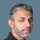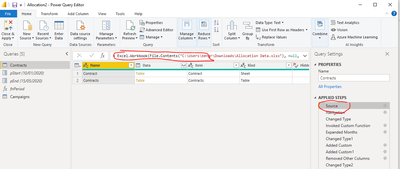cancel
Showing results for
Did you mean:## Split Amount Across Months Using Power Query

In this article, we will look at how to solve an allocation issue where you need to deal with start and end dates falling within different dates in months.

For Con-01 in the table below, the amount of 110,000 has to be divided by 291 days and multiplied by 22 days in Jan-2020, 29 days in Feb-2020, and so on, Oct-2020 will have 26 days. looks pretty simple?The challenge is that any addition of new contracts or changes in contract periods should automatically adjust the number of rows and columns, in the meantime calculating the monthly amounts correctly as explained above.

The Pivot Table below shows the desired result that we are trying to achieve here. It is giving the flexibility to analyze the above contracts in various date dimensions, even with a large number of records.Power Query Steps:

• Imported the contracts table from the Excel file (Find the Excel and the Power BI files below my signature)
• Change the data types as above if required.
• Create two parameters for the start and end dates. I named them pStart and pEnd
• The key element of this solution is the function that I created to generate a list of months and days in each month. I used the two parameters (pStart and pEnd) for the function to receive the Start and End dates of each contract.
• Create a blank query and paste the following code. I named my function as fnPeriod. The List.Generate function is a little complicated to understand but once you learn it, you will love it.

``````(pStart as date, pEnd as date) => let
Source = List.Generate(
()=> [fDate = pStart, fDay= Date.DaysInMonth(fDate)-Date.Day(fDate)+1],
each [fDate] <= pEnd,
each
let EoM = Date.EndOfMonth( Date.AddMonths([fDate],1)) in
if EoM > pEnd then
[fDate= Date.AddDays(Date.EndOfMonth([fDate]), Date.Day(pEnd)) , fDay= Date.Day(fDate)]
else
[fDate= EoM , fDay = Date.Day(fDate)]
),
ToTable = Table.FromList(Source, Splitter.SplitByNothing(), null, null, ExtraValues.Error),
ExpandTable = Table.ExpandRecordColumn(ToTable, "Column1", {"fDate", "fDay"}, {"fDate", "fDay"}),
ChangedType = Table.TransformColumnTypes(ExpandTable,{{"fDate", type date}, {"fDay", Int64.Type}})
in
ChangedType``````

• Next, I invoked the function in the Contract table passing the Start and End dates to the parameters pStart and PEnd respectively.
• Expanded the newly added column, now I have two columns giving me the month and days in each month.
• Added a custom column, “Monthly Amount” to calculate the proportionate amount for each month.
• Added another custom column "Month Year" to extract the end of the month for the dates in order to analyze by months.
• Remove unnecessary columns and uploaded the query to the data model to visualize as shown above in the matrix.

I hope you found this article useful and learned some new techniques as well

Hi @Fowmy ,

This logic is insanely useful, however I managed to find a flaw: if the start date and end date are both within the same month, and they are not the first and last day of the month, the calculation fDay is wrong. Try adding to your Allocation Data excel file a row with:

- Start 4/7/2022

- End 4/9/2022

The calculation returns 24.

As I am not very experienced with M-language, can you assist with a fix for that, if possible?

@Fowmy Your query does exactly what I want. However there is one small issue which I cannot overcome. Your source data is imported from Excel. The data I want to use is already in a Power BI table. I have saved a version of your PBIX with a table (Campaigns) in it with same data. Can you help me with amending the Source to read from the table rather Excel?PBIX Allocation2

Top Kudoed Posts
Latest Articles
Archives
Polls
What is your favorite Power BI feature release for September 2023?